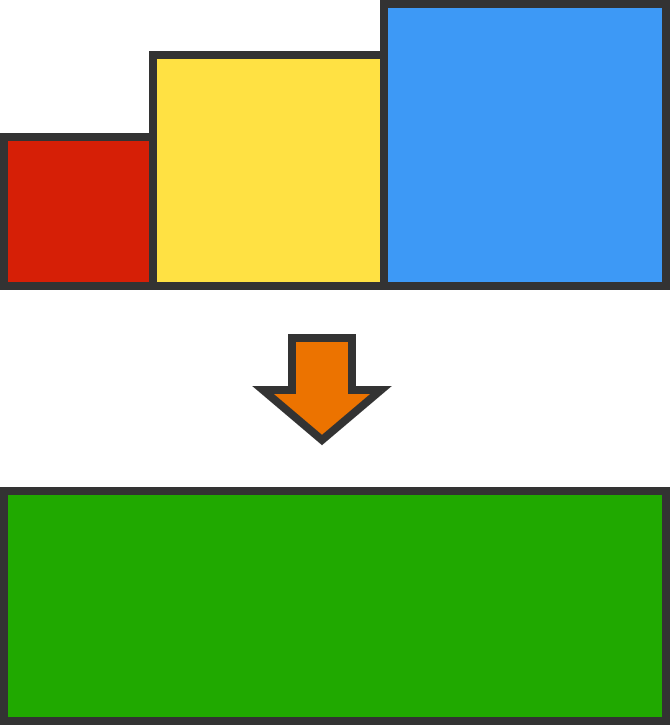# Tricking the Magician

You met a magician on a train, and after a little chat, he took out $3$ squares of different sizes, as shown below. (The figure may not be drawn to scale.)

Magician: "These squares' side lengths are distinct digits (from $1$ to $9$ inclusive) whose greatest common factor is $1$."

With a flip of his hand, he transformed the squares into one whole rectangle.Magician: "Now this rectangle's area is the same as the combined area of those $3$ squares. The width of the rectangle is equal to the sum of the $3$ squares’ side lengths, while the height of the rectangle is another distinct digit."
You: "How amazing! Can you tell me that height then?"
Magician: "No. Even if you know it, you still can’t work out the area of the rectangle."
You: "Can you at least tell me just one side length of the squares then?"
Magician: "No. Even if you know just any one square's length, you still can’t work out the area of the rectangle."
You: "Thanks! Now I know the area of the rectangle."

The magician became baffled after he had been advertently tricked to slip out a big clue.

What is the area of the rectangle?

Inspired by Digitalize This.

×